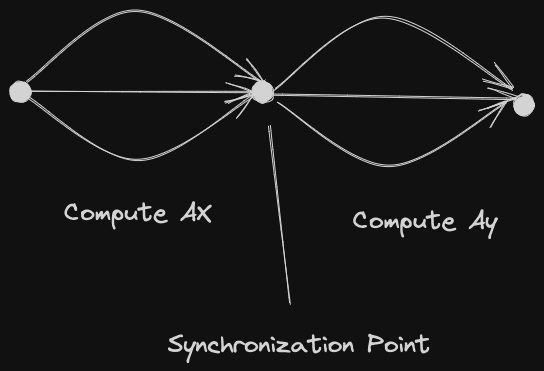Concurrency

# thread (C++) §

First intro was in CS247.

Resources

CS343

Now, I don’t get why for CS343, we don’t learn about this library. Rather, we build our own. I guess to REALLY understand how concurrency works. They do a bottom-up approach?

To use, simply include the <thread> library.

#include <thread>


### CS247 Notes §

Most CPUs in the modern day have more than one core. This can sometimes allow extraction of extra efficiency.

Example: Matrix Multiplication

Typically, compute entries in order:

In a multithreaded program: Create separate threads to perform the computations simulataneously.

void multiplyRow(int row, const vector<vector<int>>& A, const vector<int>& x, vector<int>& output) {
output[row] = 0;
for (int i=0;i<A[row].size();i++) {
output[row] += x[i] . A[row][i];
}
}

This allows us to multiply each row for our product .

vector<vector<int>> A {{1,2,3}, {4,5,6}, {7,8,9}};
vector<int> x {10,11,12};
vector<int> y{0,0,0};

for (int i=0;i<A.size();i++) {
}
t->join(); // waits, stalls the program until t has finished running.
delete t;
}
// by this point, all threads have completed - we can now use y

Why do we need ref/cref, the thread ctor implictly copies / moves its parameters.

cref, ref: create reference-wrapper object types, which can be copied / moved.

• distributed the workload. Each thread works independently on a separate part of the problem.

This is an example of an “embarrassingly parallelizable” problem.

What about synchronization? First: Compute Then compute gives us

We need to compute in entirety, before we may computeSimply:

• Spawn threads for , join them.
• Spawn new threads for , join them

But: creating threads is expensive. What if we reuse the same threads?

Let’s do a barrier example: 2 functions - f1 and f2: both must complete phase 1, then they can proceed to phase 2.

bool t1Ready = false, t2Ready = false;
void f1() {
cout << "Thread 1 Phase 1" << endl;
cout << "Thread 1 Phase 2" << endl;
}

void f2() {
cout << "Thread2 Phase 1" << endl;
cout << "Thread 2 Phase 2" << endl;

}

Why are the outputs different?

You should run this for yourself, sometimes the output will be differing. This is because the << is actually a function call. Since the threads run simultaneously, you actually don’t have the guarantee that an entire line runs alone and prints together (because there are actually two function calls in that single line).

is there a solution? Yes, use \n instead of endl.

Use std::atomic<bool> to prevent incorrect compiler optimizations with multithreaded code.

### How does it work at hardware level? §

How does it actually work in hardware?

• A hardware thread is a physical core
• A 4 core CPU can only physically supports 4 threads at once
• A software thread is created by the Operating System

One hardware thread can run many software threads. This is done time-slicing - each thread gets a few milliseconds to execute before the OS schedules another thread to run on that CPU. Since the OS switches back and forth between the threads quickly, it appears as if one CPU is doing more than one thing at once, but in reality, a core is still running only one hardware thread, which switches between many software threads.

Under the hood

So really what is happening is that a core is running only one hardware thread, but this switches between many software threads to give you the illusion that you can run THOUSANDS of threads.

But also saw this comment:

• Most new expensive chips have multiple hardware threads per core. Hyper-threading and so on have been around a fair few years now and are pervasive

Software threads are a software abstraction implemented by the (Linux) kernel:

• either the kernel runs one software thread per CPU (or hyperthread) or it fakes it with the scheduler by running a process for a bit, then a timer interrupt comes, then it switches to another process, and so on